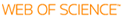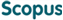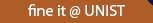### BROWSE

#### Related ResearcherLee, Jae Hwa
Flow Physics and Control Lab
Research Interests
• Turbulent Flow Physics and Control
• Incompressible and Compressible Flows
• Fluid-Structure Interaction
• Multi-Phase Flow with Heat Transfer

Turbulent boundary layers over sparsely-spaced rod-roughened walls

Cited 0 times inCited 0 times inTitle
Turbulent boundary layers over sparsely-spaced rod-roughened walls
Author
Issue Date
2015-12
Publisher
ELSEVIER SCIENCE INC
Citation
INTERNATIONAL JOURNAL OF HEAT AND FLUID FLOW, v.56, pp.16 - 27
Abstract
Direct numerical simulations (DNSs) of spatially developing turbulent boundary layers (TBLs) over sparsely-spaced two-dimensional (2D) rod-roughened walls were performed. The rod elements were periodically arranged along the streamwise direction with pitches of p(x)/k = 8, 16, 32, 64 and 128, where p(x) is the streamwise spacing of the rods, and k is the roughness height. The Reynolds number based on the momentum thickness was varied from Re-0 = 300-1400, and the height of the roughness element was k = 1.5 theta(in), where theta(in), is the momentum thickness at the inlet. The characteristics of the TBLs, such as the friction velocity, mean velocity, and Reynolds stresses over the rod-roughened walls, were examined by varying the spacing of the roughness features (8 <= p(x)/k <= 128). The outer-layer similarity between the rough and smooth walls was established for the sparsely-distributed rough walls (p(x)/k >= 32) based on the profiles of the Reynolds stresses, whereas those are not for p(x)/k = 8 and 16. Inspection of the interaction between outer-layer large-scale motions and near-wall small-scale motions using two-point amplitude modulation (AM) covariance showed that modulation effect of large-scale motions on near-wall small-scale motions was strongly disturbed over the rough wall for p(x)/k = 8 and 16. For p(x)/k >= 32, the flow that passed through the upstream roughness element transitioned to a smooth wall flow between the consecutive rods. The strong influence of the surface roughness in the outer layer for p(x)/k = 8 and 16 was attributed to large-scale erupting motions by the surface roughness, creating both upward shift of the near-wall turbulent energy and active energy production in the outer layer with little influence on the near-wall region. ess, creating both upward shift of the near-wall turbulent energy and active energy production in the outer layer with little influence on the near-wall region.
URI
https://scholarworks.unist.ac.kr/handle/201301/16425
URL
http://www.sciencedirect.com/science/article/pii/S0142727X15000715
DOI
10.1016/j.ijheatfluidflow.2015.06.006
ISSN
0142-727X
Appears in Collections:
MEN_Journal Papers
Files in This Item:
There are no files associated with this item.can give you direct access to the published full text of this article. (UNISTARs only)